# Integrated rate equations and half-life

Chemical Kinetics: Integrated rate equations and half-life

Rate law can be defined in two formats with the help of calculus. Differential Rate Law shows how the reaction rate changes with concentrations. Integrated Rate Law shows how the concentrations of species in the reaction change with time. Because the differential and integrated rate laws for a given reaction are related to each other, the experimental analysis of either of the rate laws is sufficient.

To find integrated rate law we can follow these steps.

When the two expressions are set equal, we get an expression that can be rearranged in the form of a straight line.

Zero Order reactions may exist but are relatively rare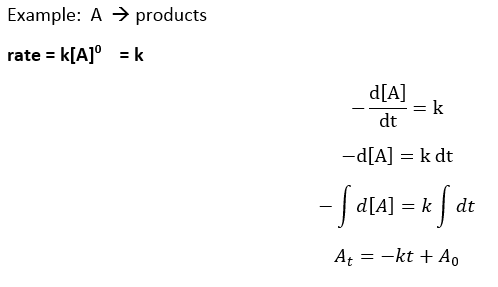Thus, a plot of [A] vs time for a 1st order reaction produces a straight line.Fig 1: Plot for 0th order reaction.

Now, consider a first-order reaction: A –> B

We already know the rate law for a 1st order reaction is

rate = k[A]

Using calculus solve the differential equation.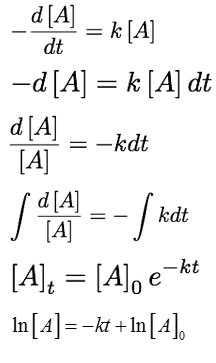[A]0 is the initial concentration of A (t=0).[A]t is the concentration of A at some time, t, during the reaction. Also, -k is the gradient of the straight-line ln[A] plotted on y-axis and t (time) plotted on x-axis and ln[A]0 being the y-intercept.

Likewise, if the concentration and time data for a reaction is given, graphing can determine the order.Fig 2: For a 1st order reaction, a plot of ln [A] vs time yields a straight line

If the plot is a straight line, then the integrated rate law equation can be used to find the rate constant, k, or the slope of the line can be calculated for the rate constant.

Half-life is the time that it takes for the reactant concentration to drop to half of its original value. It is NOT half the time it takes for all of the reactants to disappear. The shorter the half-life, the faster is the reaction.

The expression for half-life for a 1st order reaction is simplified as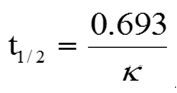The half-life for a first-order reaction is related to the reaction constant. For a first-order reaction, t1/2 does not depend on the initial concentration. Radioactive decay is a first-order reaction and the 50-50 percent for a radioactive nucleus is a useful indicator of its stability.

Alternatively, t1/2 is directly proportional to the initial concentration for a zero-order reaction,: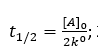Zero order process; rate = k

In zero order reaction, Half-life gets shorter as the reaction progresses and the concentration of reactants decreases.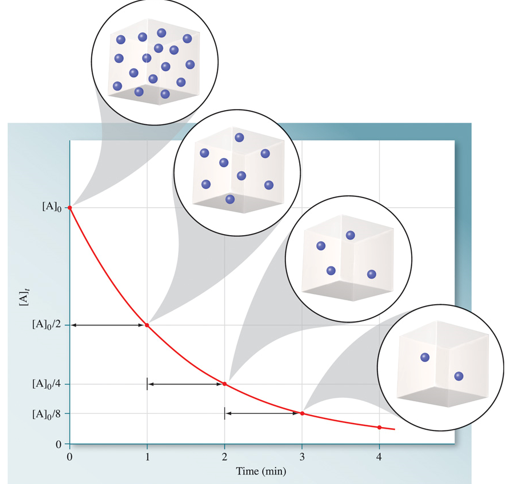Fig 2: Half-life for a 1st order reaction.

 Zero Order First Order Rate law rate = k rate = k[A] Units for k mol/L·s 1/s Half-life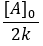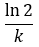Integrated rate law Straight line format [A]t = –kt + [A]0 ln[A]t = –kt + ln[A]0 Plot for straight line [A]t vs. t ln[A]t vs. t Slope, y intercept –k, [A]0 -k, ln[A]0 Plot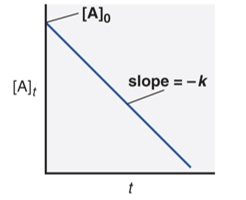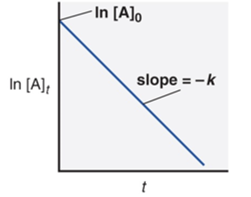Fig 3: Summary for the rate law. Here, [A] is the concentration of A at any time t and [A]0 is the concentration of A at time t=0